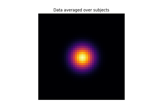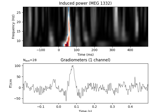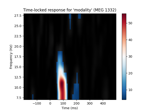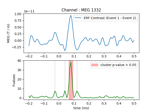# mne.stats.permutation_cluster_test¶

mne.stats.permutation_cluster_test(X, threshold=None, n_permutations=1024, tail=0, stat_fun=None, adjacency=None, n_jobs=1, seed=None, max_step=1, exclude=None, step_down_p=0, t_power=1, out_type='indices', check_disjoint=False, buffer_size=1000, verbose=None)[source]

Cluster-level statistical permutation test.

For a list of `NumPy arrays` of data, calculate some statistics corrected for multiple comparisons using permutations and cluster-level correction. Each element of the list `X` should contain the data for one group of observations (e.g., 2D arrays for time series, 3D arrays for time-frequency power values). Permutations are generated with random partitions of the data. See 1 for details.

Parameters
X`list` of `array`, shape (n_observations, p[, q])

The data to be clustered. Each array in `X` should contain the observations for one group. The first dimension of each array is the number of observations from that group; remaining dimensions comprise the size of a single observation. For example if `X = [X1, X2]` with `X1.shape = (20, 50, 4)` and `X2.shape = (17, 50, 4)`, then `X` has 2 groups with respectively 20 and 17 observations in each, and each data point is of shape `(50, 4)`. Note: that the last dimension of each element of `X` should correspond to the dimension represented in the `adjacency` parameter (e.g., spectral data should be provided as `(observations, frequencies, channels/vertices)`).

threshold

If numeric, vertices with data values more extreme than `threshold` will be used to form clusters. If threshold is `None`, an F-threshold will be chosen automatically that corresponds to a p-value of 0.05 for the given number of observations (only valid when using an F-statistic). If `threshold` is a `dict` (with keys `'start'` and `'step'`) then threshold-free cluster enhancement (TFCE) will be used (see the TFCE example and 2).

n_permutations`int`

The number of permutations to compute.

tail`int`

If tail is 1, the statistic is thresholded above threshold. If tail is -1, the statistic is thresholded below threshold. If tail is 0, the statistic is thresholded on both sides of the distribution.

stat_fun

Function called to calculate the test statistic. Must accept 1D-array as input and return a 1D array. If `None` (the default), uses `mne.stats.f_oneway()`.

Defines adjacency between locations in the data, where “locations” can be spatial vertices, frequency bins, etc. If `False`, assumes no adjacency (each location is treated as independent and unconnected). If `None`, a regular lattice adjacency is assumed, connecting each location to its neighbor(s) along the last dimension of each group `X[k]` (or the last two dimensions if `X[k]` is 2D). If `adjacency` is a matrix, it is assumed to be symmetric (only the upper triangular half is used) and must be square with dimension equal to `X[k].shape[-1]` or `X[k].shape[-1] * X[k].shape[-2]` .

n_jobs`int`

The number of jobs to run in parallel (default 1). Requires the joblib package.

seed`None` | `int` | instance of `RandomState`

If `seed` is an `int`, it will be used as a seed for `RandomState`. If `None`, the seed will be obtained from the operating system (see `RandomState` for details). Default is `None`.

max_step`int`

Maximum distance along the second dimension (typically this is the “time” axis) between samples that are considered “connected”. Only used when `connectivity` has shape (n_vertices, n_vertices).

exclude

Mask to apply to the data to exclude certain points from clustering (e.g., medial wall vertices). Should be the same shape as X. If None, no points are excluded.

step_down_p`float`

To perform a step-down-in-jumps test, pass a p-value for clusters to exclude from each successive iteration. Default is zero, perform no step-down test (since no clusters will be smaller than this value). Setting this to a reasonable value, e.g. 0.05, can increase sensitivity but costs computation time.

t_power`float`

Power to raise the statistical values (usually F-values) by before summing (sign will be retained). Note that `t_power=0` will give a count of locations in each cluster, `t_power=1` will weight each location by its statistical score.

Output format of clusters within a list. If `'mask'`, returns a list of boolean arrays, each with the same shape as the input data (or slices if the shape is 1D and adjacency is None), with `True` values indicating locations that are part of a cluster. If `'indices'`, returns a list of tuple of ndarray, where each ndarray contains the indices of locations that together form the given cluster along the given dimension. Note that for large datasets, `'indices'` may use far less memory than `'mask'`. Default is `'indices'`.

check_disjointbool

Whether to check if the connectivity matrix can be separated into disjoint sets before clustering. This may lead to faster clustering, especially if the second dimension of `X` (usually the “time” dimension) is large.

buffer_size

Block size to use when computing test statistics. This can significantly reduce memory usage when n_jobs > 1 and memory sharing between processes is enabled (see `mne.set_cache_dir()`), because `X` will be shared between processes and each process only needs to allocate space for a small block of locations at a time.

verbose

If not None, override default verbose level (see `mne.verbose()` and Logging documentation for more). If used, it should be passed as a keyword-argument only.

Returns
F_obs`array`, shape (n_tests,)

Statistic (F by default) observed for all variables.

clusters`list`

List type defined by out_type above.

cluster_pv`array`

P-value for each cluster.

H0`array`, shape (n_permutations,)

Max cluster level stats observed under permutation.

References

1

Eric Maris and Robert Oostenveld. Nonparametric statistical testing of EEG- and MEG-data. Journal of Neuroscience Methods, 164(1):177–190, 2007. doi:10.1016/j.jneumeth.2007.03.024.

2

Stephen M. Smith and Thomas E. Nichols. Threshold-free cluster enhancement: addressing problems of smoothing, threshold dependence and localisation in cluster inference. NeuroImage, 44(1):83–98, 2009. doi:10.1016/j.neuroimage.2008.03.061.

## Examples using `mne.stats.permutation_cluster_test`¶